Clouds!

Gene Pang

Introduction

The purpose of this project is to simulate the dynamic nature of clouds and produce an animation. The movement of clouds is an interesting phenomemenon that appears complicated. Here is the original proposal. Here is a motivating video of clouds.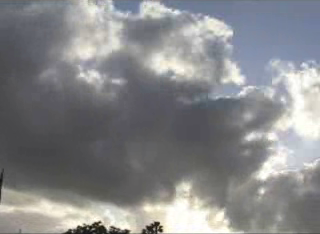In order to achieve this animation, 2 papers on cloud simulations were used. Dobashi's paper "A Simple, Efficient Method for Realistic Animation of Clouds" and Miyazaki's paper "A Method for Modeling Clouds Based on Atmospheric Fluid Dynamics" were used to learn about the computer simulation aspects of clouds. The Dobashi paper uses cellular automaton to simulate the formation, dynamics, and extincting of clouds. The Miyazaki paper extends the Dobashi concept and uses coupled map lattices(cmls) to do the simulation. I have tried both techniques outlined in both papers, and ultimately chose to use the cellular automaton in the Dobashi paper. This decision was made because I was unsuccessful in determining all the parameters for the cmls.

Cellular Automaton

In order to simulate clouds, a 3d cellular automaton is used. A 3d cellular automaton is a collection of 3d cells, or voxels, which hold binary values and evolve to different configurations depending on the neighboring cells. For each cell, several state variables are stored. hum (water vapor), cld(clouds), act(phase transition), pext(probability of extinction), pact(probability of phase activation), phum(probability of vapor) are part of the state for each cell. The probabilities are all floating point values and the other state variables are just bit flags. The simulation space is set up as a grid of nx * ny * nz cells. The rules for the binary state variables are:

• hum(x, y, z, t+1) = hum(x, y, z, t) && ~act(x, y, z, t)
• cld(x, y, z, t+1) = cld(x, y, z, t) || act(x, y, z, t)
• act(x, y, z, t+1) = ~act(x, y, z, t) && hum(x, y, z, t) && fact(x, y, z)
• fact(x, y, z) = act(x+1, y, z) || act(x, y+1, z) || act(x, y, z+1) || act(x-1, y, z) || act(x, y-1, z) || act(x, y, z-1) || act(x+2, y, z) || act(x-2, y, z) || act(x, y+2, z) || act(x, y-2, z) || act(x, y, z-2)

The intuition is that clouds form when the phase activation is on. The phase activation occurs when the neighboring cells are activated and there is water vapor. The z+2 term is not included in the neighbors, because clouds tend to grow upwards. If the phase transition happens, then the water vapor turns into clouds so the humidity goes away and the cloud appears.

Cloud Extinction / Creation

Along with the automaton transition rules, additional rules have to be run to allow for cloud extinction and formation. At each cell, there are 3 probabilities associated with it; pext, phum, pact. The pext is the probability that the cloud in that cell will die. The pext and pact are the probabilities that the cell will create a cloud or humidity. The rules for the probabililities are:

• if (rand() < pext(x, y, z, t)) then cld(x, y, z, t+1) = 0
• if (rand() < phum(x, y, z, t)) then hum(x, y, z, t+1) = 1
• if (rand() < pact(x, y, z, t)) then act(x, y, z, t+1) = 1

Each cell has the 3 probabilities and the values control the animation. The probabilities are distributed throughout the simulation space as a set of ellipsoids.

Ellipsoids

Ellipsoids are used to control the animation. The ellipsoids determine the distribution and values of the probabilities of pext, phum, and pact in the simulation space. For pext, the center of the ellipsoid is the lowest value, and outside the ellipsoid is the largest value. For phum and pact, the centers have the highest values, and the cells outside of the ellipsoid have the lowest values. This follows from the fact that cloud extinction happens more frequently at the edges. The probability distribution is just a piecewise linear function of the normalized distance from the center of the ellipsoid.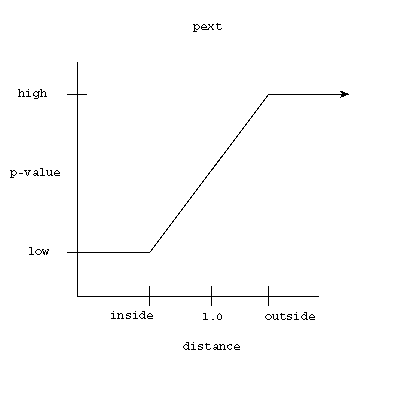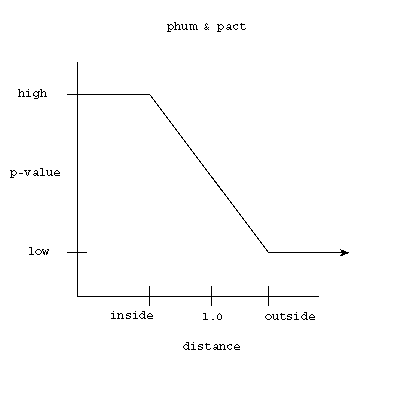Wind

To simulate wind, a random vector of integer values 1 and 2 is generated with perlin noise. Each value is the wind speed for a particular height of the simulation space. For each time step, all the state values, probabilities and ellipsoid centers are moved by the wind speed.

Rendering

Before outputting the density data (the cld state variable), filters have to be performed, since all the state variables are binary values 1 or 0. A gaussian filter is applied to the 3d cellular automaton. Also, a gaussian filter between time steps is applied, to smooth out the animation. At each timestep, the density volume is output into a file that pbrt can read. After many frames are generated, a script calls pbrt on all the input files.

Results

XviD codec is required to play the movies.

clouds in mountains (50 ellipsoids)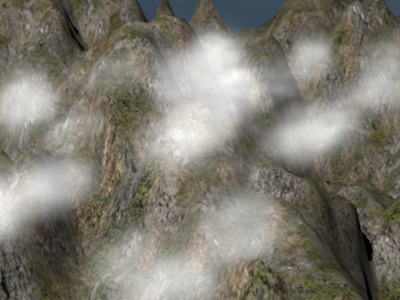clouds in mountains (80 ellipsoids)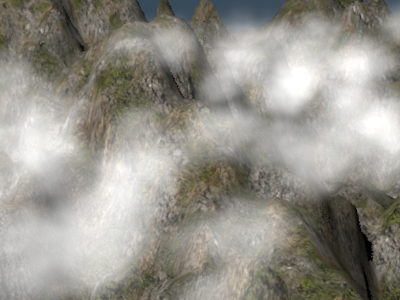clouds in a room (50 ellispoids)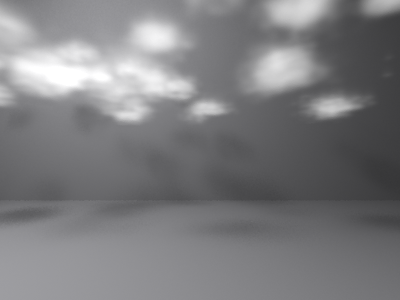References

Y.Dobashi, K.Kaneda, H.Yamashita, T.Okita, T.Nishita, "A Simple, Efficient Method for Realistic Animation of Clouds," Proc. of SIGGRAPH2000

Ryo Miyazaki , Satoru Yoshida , Tomoyuki Nishita , Yoshinori Dobashi, A Method for Modeling Clouds Based on Atmospheric Fluid Dynamics, Proceedings of the 9th Pacific Conference on Computer Graphics and Applications, p.363, October 16-18, 2001